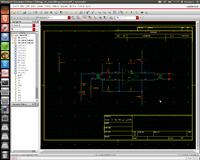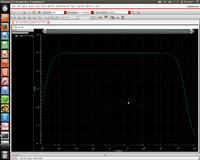# [SOLVED]Measure close loop gain op fully op amp

Status
Not open for further replies.

#### Analog Ground

##### Full Member level 4Along with your frequency plots of the individual signals, add a plot of (inp-inn)/(outp-outn). Your simulator should be able to plot this function.

#### LvWAlong with your frequency plots of the individual signals, add a plot of (inp-inn)/(outp-outn). Your simulator should be able to plot this function.
I would suggest tp plot the invers of the given expression, since the gain is defined as output/input.

#### Analog Ground

##### Full Member level 4I would suggest tp plot the invers of the given expression, since the gain is defined as output/input.

Yes, I made a silly error. (outp-outn)/(inp-inn)

#### tompham

##### Full Member level 2Thanks Analog Ground and LvW for your suggestion. I make a new schematic to run with cadence spectre (attached picture). I plot differential gain vesus frequency (close loop gain) , the result from spectre is not what i expect. From formular for close loop gain Acl = Aol/ (1 + B*Aol) B is feed back factor
From schematic B = C2 / (C1 + C2 + Cin) where Cin is total op amp input capacitor (Cin = 0.7pF in my simulation)

So the feedback factor B = 1 / (1 + 3 + 0.7) = 0.2
My open loop gain is 54dB, and from Acl = Aol/ (1 + B*Aol) ---> Acl = 1/B
---> Acl = 1/0.2 = 5
The spectre simulation shows Acl = 3 only
I thinking the open loop gain is a litle low but this is not the case because 54dB is OK.
Anyone has any idea to fix this problem please let me know. Thanks a lot- - - Updated - - -

I make a mistake in caculate Aol:

Aol ~Cin/Cfb = 3/1 = 3. The simulation is correct

Status
Not open for further replies.